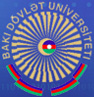## BSU professor’s paper has published in high-impact journal

13/06/2020

The scientific article entitled “Global bifurcation of positive solutions from zero in nonlinearizable elliptic problems with indefinite weight” by Ziyatkhan Aliyev, Professor at  the Department of Mathematical Analysis of BSU was released in the Journal of Mathematical Analysis and Applications.

This paper is devoted to the study of global bifurcation of solutions from zero of some nonlinearizable eigenvalue problems for second order elliptic partial differential equations with indefinite weight. The existence of global continua of solutions emanating from bifurcation intervals surrounding the trivial solutions corresponding to the principal eigenvalues of the linear problem obtained by setting the nonlinear term equal to zero and contained in the classes of positive and negative functions is shown.
The Journal of Mathematical Analysis and Applications is an academic journal in mathematics, specializing in mathematical analysis and related topics in applied mathematics. For most years since 1997 it has been ranked by SCImago Journal Rank as among the top  journals in its topic areas.